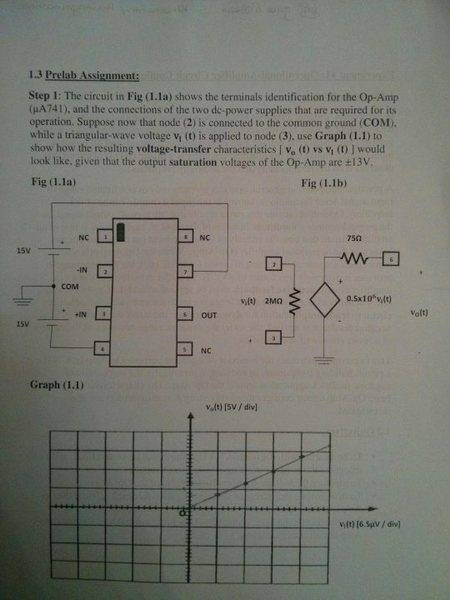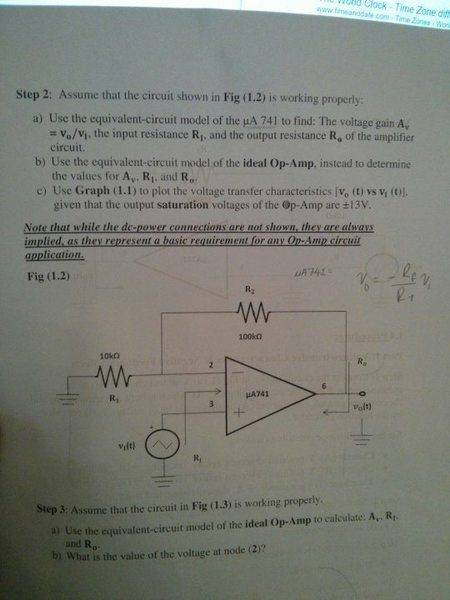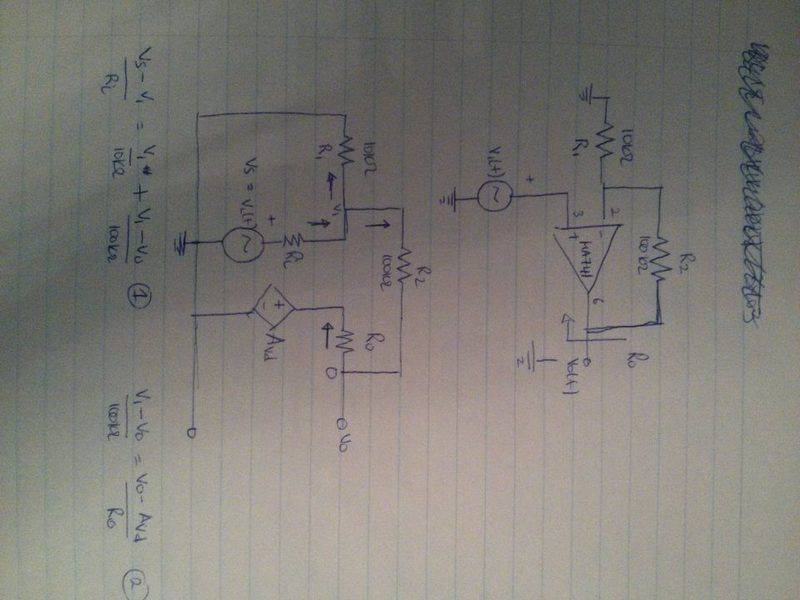# Find the voltage gain, input and output resistance amplifier

I am having a difficult time solving the question in Step 2 of the attachment. The question is "Use the equivalent-circuit model of the uA 741 to find: The voltage gain Av=vo/v1, the input resistance R1, and the output resistance Ro of the amplifier circuit.Attempt at solution:

I used the equivalent circuit model of the uA 741 to create two equations. My understanding is that I solve for V1 in the first equations and substitute it into the second equation to get the voltage gain Av=Vo/V1, where Vs is V1 in my equations. The problem I am having is how am I supposed to find the input and output resistance, where I don't know V1,donpacino
Gold Member
A few things to note...

The voltage gain Av and the op amp gain Avo are two very different things.

What is the input/output resistance of an ideal Op-amp (not this circuit, just the op amp).
What is the gain of an ideal op amp?
To find input and output resistances you zero out all independent sources.

Also the dependent source on the output of your op amp should be
AVD=Avo*(Vin+ - Vin-)

another question... with negative feedback in an ideal amplifier, what is the relationship between Vin- and Vin+

•sugz
A few things to note...

The voltage gain Av and the op amp gain Avo are two very different things.

What is the input/output resistance of an ideal Op-amp (not this circuit, just the op amp).
What is the gain of an ideal op amp?
To find input and output resistances you zero out all independent sources.

Also the dependent source on the output of your op amp should be
AVD=Avo*(Vin+ - Vin-)

another question... with negative feedback in an ideal amplifier, what is the relationship between Vin- and Vin+
The input and output resistance of the op amp itself is 2 megaohms and 75 ohms, respectively. The gain of the op amp 0.5x10^6. This is the second week of this course, where we haven't learnt much of this. I am confused if I am missing something. I am not sure I quite follow what you mean when you say to "to find the input and output resistance you zero out all independent sources. Please help!

donpacino
Gold Member
The input and output resistance of the op amp itself is 2 megaohms and 75 ohms, respectively. The gain of the op amp 0.5x10^6. This is the second week of this course, where we haven't learnt much of this. I am confused if I am missing something. I am not sure I quite follow what you mean when you say to "to find the input and output resistance you zero out all independent sources. Please help!
to find the input and output resistances set all independant sources (read Vs) to zero..
so set Vs=0

to find the input and output resistances set all independant sources (read Vs) to zero..
so set Vs=0
Is the input and output resistance being asked in part (a) different from the input and output resistance of the op amp itself (i.e. 2 MegaOhms and 75 Ohms, respectively)?

NascentOxygen
Staff Emeritus
Is the input and output resistance being asked in part (a) different from the input and output resistance of the op amp itself (i.e. 2 MegaOhms and 75 Ohms, respectively)?
For part (a) you must analyse the circuit you have drawn. As donpacino says, you set Vs to zero, and apply a voltage to the circuit's output. Determine the current that flows from this, and their quotient tells you the circuit's output impedance.

donpacino
Gold Member
Is the input and output resistance being asked in part (a) different from the input and output resistance of the op amp itself (i.e. 2 MegaOhms and 75 Ohms, respectively)?
yes they are different. remember that there are two resistors in the circuit in addition to the op amp

yes they are different. remember that there are two resistors in the circuit in addition to the op amp
Most important: These two resistors provide negative feedback!
The ouput resistance Ro of the opamp is reduced drastically using feedback .
The resulting output resistance is r,out=Ro/(1+LG)
with Loop gain LG= Aol*beta (beta: feedback factor).